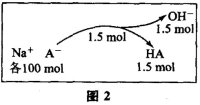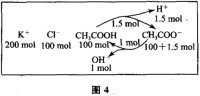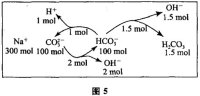#学习小专题

## 基于模型思想的离子浓度大小比较

1．单一溶液离子浓度比较：

A．c(CH3COOH) ＞ c(H+) ＞ c(CH3COO) ＞ c(OH)

B．c(CH3COOH) ＞ c(CH3COO) ＞ c(H+) ＞ c(OH)

C．c(CH3COOH) ＞ c(CH3COO) ＝ c(H+) ＞ c(OH)

D．c(CH3COOH) ＞ c(CH3COO) ＞ c(OH) ＞c(H+

A．c(Na) ＞ c(A) ＞ c(H+) ＞ c(OH B．c(Na) ＞ c(OH) ＞ c(A) ＞ c(H+)

C．c(Na) + c(OH) = c(A) +  c(H+    D．c(Na) + c(H+) = c(A)+ c(OH)A．c(Na+) ＞ c(HCO3) ＞ c(H+) ＞ c(OH)

B．c(Na+)＋c(H+) = c(HCO3)＋c(OH)＋2 c(CO32−)

C．c(Na+) = c(HCO3) ＞ c(OH) ＞c(H+)

D．c(Na+) = c(HCO3) ＋ c(H2CO3) ＋2c(CO32−)

2．混合溶液离子浓度比较

A．c(CH3COO) = c(Cl) = c(H+) ＞ c(CH3COOH)

B．c(CH3COO) = c(Cl) ＞ c(CH3COOH) ＞ c(H+)

C．c(CH3COO) ＞ c(Cl) ＞ c(H+) ＞ c(CH3COOH)

D．c(CH3COO) ＞ c(Cl) ＞ c(CH3COOH) ＞ c(H+)3. 综合训练

A. 在0.1 mol・L1NaHCO3溶液中：c(Na)＞c(HCO3)＞c(CO32)＞c(H2CO3)

B. 在0.1 mol・L1Na2CO3溶液中：c(OH)－c(H)＝c(HCO3) + 2c(H2CO3)

C. 向0.2 mol・L1NaHCO3溶液中加入等体积0.1 mol・L1NaOH溶液：

c(CO32)＞c(HCO3)＞c(OH)＞c(H)

D. 常温下，CH3COONa和CH3COOH混合溶液[pH＝7，c(Na)＝0.1 mol・L1]：

c(Na)＝c(CH3COO)＞c(CH3COOH)＞c(H)＝c(OH)（本文发表于北京师范大学《高中数理化》2012年第2期下）

(责任编辑：化学自习室)

------分隔线----------------------------

------分隔线----------------------------

 如何计算多元弱酸溶液中的粒子浓 根据图像分析微粒浓度问题专练 定量分析碳酸氢钠溶液中微粒浓度 如何比较强碱弱酸酸式盐溶液中的 选择题解题策略与技巧之离（粒） 专项突破：粒子浓度大小比较及曲 第十三讲：水溶液中的平衡体系（ 用好“四点”突破图像类溶液中微 乐乐课堂：盐类水解反应的利用 常见盐溶液中离子浓度的精准算法 2008～2017江苏高考离子浓度比较 微课视频：溶液中粒子浓度大小比 微课视频：离子浓度大小比较 电解质溶液中微粒浓度大小比较的 溶液中离子浓度大小比较的常见错 溶液中离子浓度大小的比较及其规 电解质溶液稀释时离子浓度的变化 离子浓度大小比较一、二、三、四• 全部评论（0
还没有评论，快来抢沙发吧！